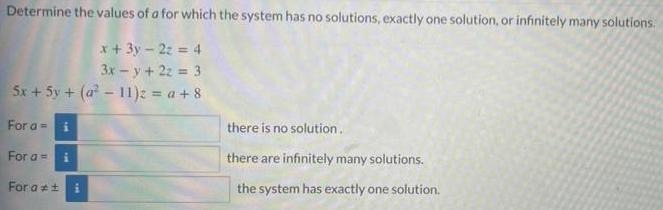Question:

# Determine the values of a for which the system has no solutions, exactly one solution, or infinitely many solutions. x + 3y - 2z = 4 3x-y + 2z = 3 5x + 5y + (a²-11)2 = a +8 For a = i For a = i For a #± there is no solution. there are infinitely many

Last updated: 8/15/2022Determine the values of a for which the system has no solutions, exactly one solution, or infinitely many solutions. x + 3y - 2z = 4 3x - y + 2z = 3 5x + 5y + (a²-11)z = a +8